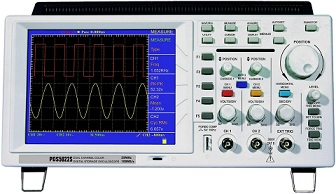## Uses of Cathode Ray Oscilloscope:

Uses of Cathode Ray Oscilloscope is probably the most versatile tool for the development of electronic circuits and systems.### Purpose of Oscilloscope:

The Uses of Cathode Ray Oscilloscope allows the amplitude of electrical signals, whether they are voltage, current, or power, to be displayed as a function of time.

The CRO depends on the movement of an electron beam, which is bombarded (impinged) on a screen coated with a fluorescent material, to produce a visible spot. If the electron beam is deflected on both the conventional axes, i.e. X-axis and Y-axis, a two-dimensional display is produced.

The beam is deflected at a constant rate relative of time along the X-axis and is deflected along the Y-axis in response to an stimulus, such as a voltage. This produces a time-dependent variation of the input voltage.

The oscilloscope is basically an electron beam voltmeter. The heart of the oscilloscope is the Cathode Ray Tube (CRT) which makes the applied signal visible by the deflection of a thin beam of electrons. Since the electron has practically no weight, and hence no inertia, therefore the beam of electrons can be moved to follow waveforms varying at a rate of millions of times/second. Thus, the electron beam faithfully follows rapid variations in signal voltage and traces a visible path on the CRT screen. In this way, rapid variations, pulsations or transients are reproduced and the operator can observe the waveform as well as measure amplitude at any instant of time.

Since it is completely electronic in nature, the oscilloscope can reproduce HF waves which are too fast for electro mechanical devices to follow. Thus, the oscilloscope has simplified many tests and measurements. It can also be used in any field where a parameter can be converted into a proportional voltage for observation, e.g. meteorology, biology, and medicine.

### Uses of Oscilloscope:

Uses of Cathode Ray Oscilloscope is thus a kind of voltmeter which uses beam instead of a pointer, and kind of recorder which uses an electron beam instead of a pen.